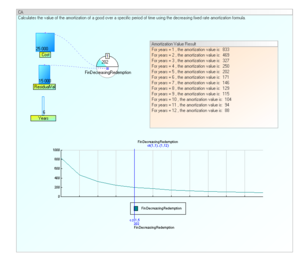Working with ... Svalue()// returns a stock valuey= Svalue("stock")y= Svalue("stock",c,t)y= Svalue("stock",False,nbt) Svalue (Stock_name)Svalue (Stock_name,Cycle,Time)Svalue (Stock_name,False,nbTime)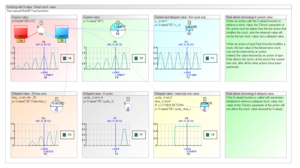Fvalue() //Returns a flow valuey= Fvalue("Flow")y= Fvalue("Flow",c,t)y= Fvalue("Flow",False, nbt) Fvalue ("Flow_name")Fvalue ("Flow_name",Cycle,Time)Fvalue ("Flow_name",False,nbTime)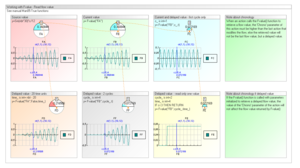Mvalue() //Returns a mirror stock value y= Mvalue("mirror_stock")y= Mvalue("mirror_stock",c,t)y= Mvalue("mirror_stock",False,nbt) Mvalue ("mirror_stock_name")Mvalue ("mirror_stock_name",Cycle,Time)Mvalue ("mirror_stock_name",False,nbTime)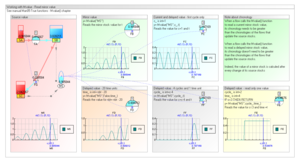In this model there is two parts: Part 1 is calculated for all cycles Part2 is calculed for cycles 25 to 30, because temporal parameters 'Cycle' (filtering cycle) of its actions are (25-30)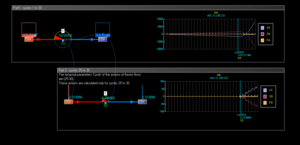The flow 'DefaultCycle' initializes the default cycle for the model. The 3 following flows have floating cycle type, temporal parameters are: start =1 , repeat = 3 start =2 , repeat = 3 start =3 , repeat = 3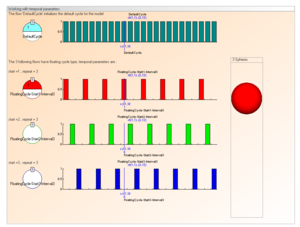The function ReStartAt() allows the computation to restart at a given time unit.In this example, the model is compiled n times until the value of the stock "SB" is equal to the value of the stock "SD".The value of the stock "SD"  is function of the values of the flows "FA" and "FB". //Only for t=12, see temporal parameter//Check condition IF Svalue("SB")=Svalue("SD") THEN RETURN //Condition not ok//We ReStart at cycle=1 and time=1, max restart = 5 c_restart,t_restart,Max_passing are intc_restart=1;t_restart=1;Max_passing=5ReStartAt(c_restart,t_restart,Max_passing)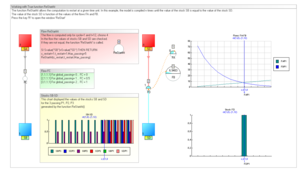Example using the FinRepayVal() Windev function (copyright PcSoft) that returns the amount of each regular payment for an investment with fixed interest Rate and fixed payment.Loan,Term,Rate,AmountMons are currenciesLoan= Svalue("Loan")Term= Svalue("Term of the loan in months")Rate= Svalue("Annual loan interest rate")AmountMons=FinRepayVal(-Loan,Term,Rate/12)Example using the FinDecreasingRedemption() Windev function (copyright PcSoft) //Value of a fixed rate amortization//The following code return an amortization value.//The acquisition cost and the residual value of the good, the amortization length,//the period over which the amortization must be calculated and the number of months//Variable declarationAmortizationValRes is a currency//amortization value for the given periodCost is currency//acquisition cost of the goodResidualVal is currency//residual value of the good after the amortizationyears is real //number of periods during which the good is amortizePeriod is int// Optional integer, period over which the amortization must be calculated (1 by default).NbMonth is int// Optional integer, number of months in the first amortization year (1 by default). Cost= Svalue("Cost")// 1000ResidualVal= Svalue("ResidualVal")// 100years= nbt//Svalue("Years")// 10Period=1NbMonth=1 //Calculate the amortization valueAmortizationValRes = FinDecreasingRedemption(Cost, ResidualVal, years, Period, NbMonth)AmortizationValRes=Round(AmortizationValRes,0)IF AmortizationValRes<>0    // Display result    y=AmortizationValRes    Aresult(2,"For years =",years,", the amortization value is: ",AmortizationValRes)ELSE    IF AmortizationValRes = 0        IF FinError = 0            Aresult(2,"The amortization value is Null")        ELSE            // Display error message            Aresult(2,ErrorInfo(errMessage))        END    ENDEND